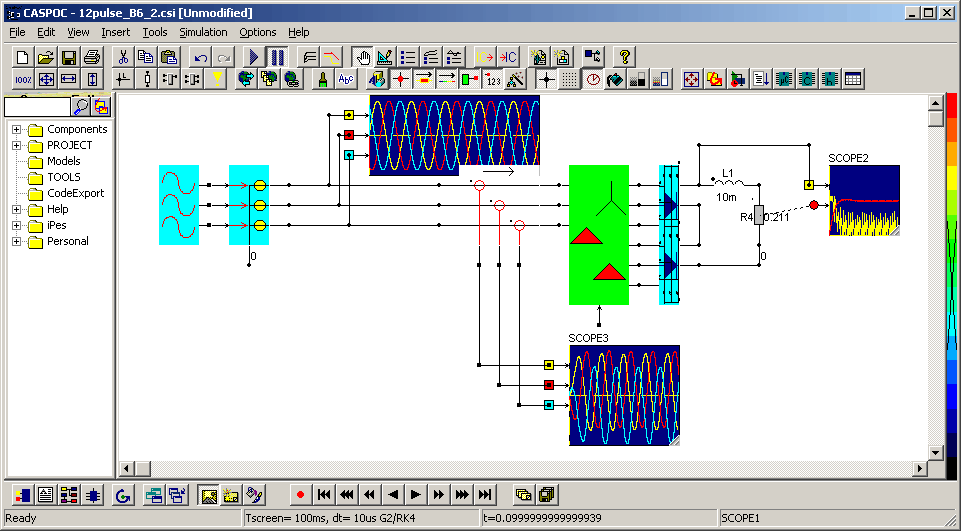• Introduction
• What is in this manual
• What is Caspoc
• User interface
• Introduction
• Starting
• Simulation
• Editing
• Viewing and printing
• Getting Started
• Basic editing
• Simulation in the time domain
• Basic User Interface Topics
• Editing
• Simulation
• Viewing
• Library
• Reports
• Project management
• Circuit and Block Diagram Components
• Introduction
• Cscript and user defined functions
• Component parameters
• Modeling Topics
• Introduction
• Power Electronics
• Semiconductors
• Electrical Machines
• Electrical drives
• Power Systems
• Mechanical Systems
• Thermal Systems
• Magnetic Circuits
• Green Energy
• Coupling to FEM
• Experimenter
• Analog hardware description language
• Embedded C code Export
• Coupling to Spice
• Small Signal Analysis
• Matlab coupling
• Tips and tricks
• Appendices

## Power Transformers.

Power transformers are either single phase or three phase. The single phase transformers always have a primairy to secondairy winding ratio for voltage and current. Three phase transformers are build in various ways. Three leg, five leg and shell type transformers have a more complicated magnetic circuit, because of the coupling between the phases.

There are three methods for modeling power transformers.

• Coupled controlled sources

The method of coupled controlled sources is applicable to single phase transformers, but is difficult to employ for three phase systems, because of the many connections.

• Coupled inductors

The method of coupled inductors is applicable to both single and three phase transformers. The problem is mainly reduced to obtaining the correct circuit parameters.

• Magnetic circuit

The magnetic circuit solves all of the above problems, because here the magnetic circuit is modeled as well as the number of windings. The magnetic circuit can either be build inside a FEM package, or modeled using an equivalent magnetic circuit

In the library transformers there are ideal, single phase and three-phase transformers. The parameters for these blocksare the inductance and coupling between the phases. The example below shows a twelve pulse DC current link.Using the components from the magnetic library, any transformer configuration can be build. Since all windings can be placed in series and parallel, any type like wye, delta or zig-zag connection can be designed. Here the number of windings and the size and material properties of the magnetic circuit are the parameters. The example below shows a wye-wye connection using magnetic components.